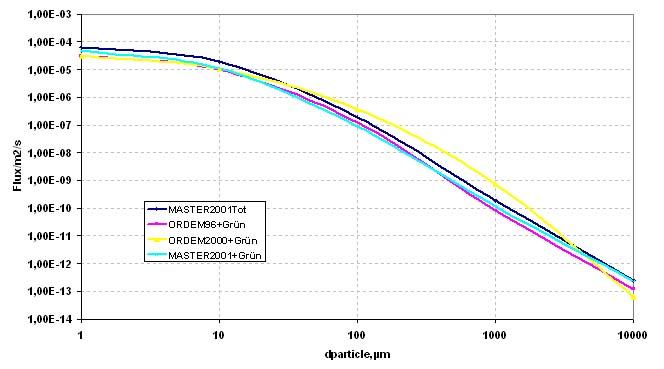## MODELS

Several models are currently used to assess the Meteoroid and Debris Environment (e.g. Sdunus,1999) These models are based mainly on results from space based and ground based observations. Five widely used models are briefly described below, more details are given in the Technical Note TN4 and references herein.

The following figure compares the fluxes resulting from the different models; they are quite similar excepted ORDEM 2000 which is a little higher than the others.Figure 2: Comparison of cumulative fluxes resulting from different environmental models

### Meteoroid Model

The meteoroid model developed by Grün et al.(Grün 1985) expresses the averaged flux resulting from meteoroids in terms of integral flux F(>m) (impacts per m2 and year resulting from particles of mass m and larger) impacting a randomly oriented plate under a viewing angle of 2pi in the ecliptic plane at 1 AU. Data come mainly from the analysis of impact craters on lunar samples. The Grün model assumes an isotropic flux environment and does not give directional information, it is given by the following simple equation:

F(m) = c0 { (c1 m0.306 + c2)-4.38 + c3 (m + c4 m2 + c5 m4)-0.36 + c6 (m + c7 m2)-0.85 }

• m is the mass in grams, c0 to c7 are constants
• c0 = 3.156 × 10E7; c1 = 2.2 × 10E3
• c2 = 15; c3 = 1.3 × 10E-9
• c4 = 10E11; c5 = 10E27
• c6 = 1.3 10E-16; c7 = 10E6

The model is valid for particles with a mass larger than 10E-18 g. The derivation of the Grün flux required an assumed meteoroid mean speed of 20 km/s.

### ESA MASTER 2001

MASTER (Meteoroid and Space Debris Terrestrial Environment Reference Model) relates to the group of so called semi-deterministic models. The purpose of MASTER is the characterization of the natural and the man-made particulate environment of the earth, and the fast and simple evaluation of the resulting effects on space missions. The model is based on semi-deterministic analysis and prediction techniques, supported by data from a number of fragmentation experiments and compilation of the current debris sources. For the meteoroids MASTER2001 used the model developed by Divine and Staubach (Divine, 1993, Staubach, 1996). Divine developed a mathematical model using measurements of interplanetary dust to determine the orbital distribution of particles. The MASTER model offers a full three dimensional description of the terrestrial debris distribution reaching from LEO (r > 6564 km) up to the GEO region (r < 45164 km). Due to the semi-deterministic background the software developed for MASTER is able to trace back the characteristics (source, orbit, mass, size) of all contributing objects.

### NASA ORDEM 96

This debris model (Kessler et al. 1996) is restricted to circular target orbits for altitudes lower than 2000 km. It gives directional flux information and is valid for particle diameters down to 10 µm. It discriminates by particle sources terms as: intact satellites, large and small fragments, paint flakes, Al2O3 particles, NaK droplets. It takes into account elliptical orbits. The model approximates the orbital debris environment by a limited number of representatives orbits (six inclination bands and two eccentricity families), each with its own number distribution as a function of size, altitude and eccentricity family, then computes the flux on a spacecraft. The functional forms used to represent the number of particles, as a function of both size and altitude result from either theoretical or experimental considerations (see Kessler et al. 1996).

### NASA ORDEM 2000

The NASA ORDEM2000 model supersede the previous model ORDEM96 (Liou et al 2002). This engineering model provides an accurate assessment of the orbital debris as a function of altitude, latitude and debris size. It is based on debris populations consistent with this requirement. It gives a complete description of the LEO environment in terms of debris flux onto spacecraft surfaces, for debris size larger than 10 µm and altitude lower than 2000 km.

Ten data sources form the basic database of ORDEM2000: SSN catalog, Haystack and Haystack auxiliary radar data (HAX), Goldstone radar data, Impact measurements from the Long-Duration Exposure Facility (LDEF), Hubble Space Telescope Solar Array (HST-SA) impact data (first retrieval), European Retrievable Carrier (EuReCa) impact data, Space Shuttle window and radiator impact data, Space Flyer Unit data, Mir impact data.

The model is then based on five pre-calculated debris populations. They correspond to objects of five different size thresholds: 10 µm and greater, 100 µm and greater, 1 cm and greater, 1 cm and greater, 10 cm and greater and 1 m and greater.